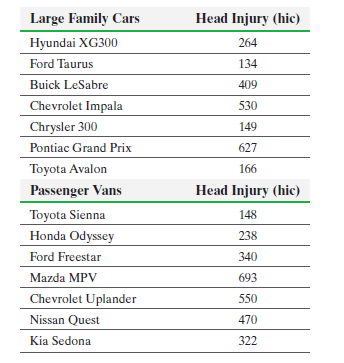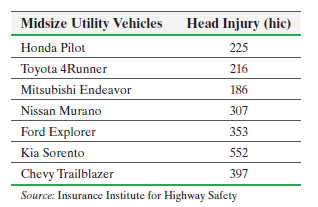×
Get Full Access to Statistics: Informed Decisions Using Data - 5 Edition - Chapter 13.1 - Problem 20
Get Full Access to Statistics: Informed Decisions Using Data - 5 Edition - Chapter 13.1 - Problem 20

×

# ?Crash Data The Insurance Institute for Highway Safety conducts experiments in which cars are crashed into a fixed barrier at 40 mph. The barrier’s defISBN: 9780134133539 240

## Solution for problem 20 Chapter 13.1

Statistics: Informed Decisions Using Data | 5th Edition

• Textbook Solutions
• 2901 Step-by-step solutions solved by professors and subject experts
• Get 24/7 help from StudySoup virtual teaching assistantsStatistics: Informed Decisions Using Data | 5th Edition

4 5 1 386 Reviews
11
1
Problem 20

Crash Data The Insurance Institute for Highway Safety conducts experiments in which cars are crashed into a fixed barrier at 40 mph. The barrier’s deformable face is made of aluminum honeycomb, which makes the forces in the test similar to those involved in a frontal offset crash between two vehicles of the same weight, each going just less than 40 mph. Suppose you want to know if the mean head injury resulting from this offset crash is the same for large family cars, passenger vans, and midsize utility vehicles. The researcher wants to determine if the means for head injury for each class of vehicle are different. The following data were collected from the institute’s study.(a) State the null and alternative hypotheses.

(b) Verify that the requirements to use the one-way ANOVA procedure are satisfied. Normal

probability plots indicate that the sample data come from normal populations.

(c) Test the hypothesis that the mean head injury for each vehicle type is the same at the a = 0.01 level of significance.

(d) Draw boxplots of the three vehicle types to support the analytic results obtained in part (c).

Step-by-Step Solution:

Step 1 of 5) Crash Data The Insurance Institute for Highway Safety conducts experiments in which cars are crashed into a fixed barrier at 40 mph. The barrier’s deformable face is made of aluminum honeycomb, which makes the forces in the test similar to those involved in a frontal offset crash between two vehicles of the same weight, each going just less than 40 mph. Suppose you want to know if the mean head injury resulting from this offset crash is the same for large family cars, passenger vans, and midsize utility vehicles. The researcher wants to determine if the means for head injury for each class of vehicle are different. The following data were collected from the institute’s study. (a) State the null and alternative hypotheses. (b) Verify that the requirements to use the one-way ANOVA procedure are satisfied. Normal probability plots indicate that the sample data come from normal populations. (c) Test the hypothesis that the mean head injury for each vehicle type is the same at the a = 0.01 level of significance. (d) Draw boxplots of the three vehicle types to support the analytic results obtained in part (c).

Step 2 of 2

## Discover and learn what students are asking

Calculus: Early Transcendental Functions : Differential Equations: Separation of Variables
?In Exercises 1-14, find the general solution of the differential equation. $$\frac{d r}{d s}=0.75 r$$

Unlock Textbook Solution